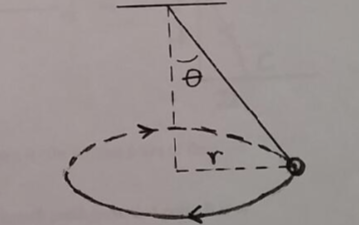# Problem: A ball of mass m = 1.30 kg is attached to a massless rope and swung in a horizontal circle of radius r = 1.70 m, as shown in the figure. The rope makes a constant angle θ with the vertical as the ball swings around the circle, and the ball makes one revolution around the circle in a time Δt = 3.00 s.Determine the ball's speed as it moves around the circle.

###### FREE Expert Solution
84% (253 ratings)
###### Problem Details

A ball of mass m = 1.30 kg is attached to a massless rope and swung in a horizontal circle of radius r = 1.70 m, as shown in the figure. The rope makes a constant angle θ with the vertical as the ball swings around the circle, and the ball makes one revolution around the circle in a time Δt = 3.00 s.

Determine the ball's speed as it moves around the circle.